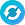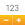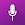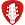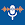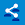# Space Math - Times tables & multiplication games

by UXApps Ltd
100,000+ installs

Android application Space Math - Times tables & multiplication games developed by is listed under category Game Educational. According to Google Play Space Math - Times tables & multiplication games achieved more than 100,000 installs. Space Math - Times tables & multiplication games currently has 1,408 ratings with average rating value of 4.13. The current percentage of ratings achieved in last 30 days is 0.82%, percentage of ratings achieved in last 60 days is 1.93%. Space Math - Times tables & multiplication games has the current market position #109177 by number of ratings. A sample of the market history data for Space Math - Times tables & multiplication games can be found below. Last update on 2022-09-04.

Title: Space Math - Times tables & multiplication games UXApps Ltd Game Educational Free Android
Total ratings: 1408 0.82% 1.93% 4.13
Installs (achieved): 100,000+ 241,095
 5 star ratings: 896 234 64 0 213

2021-08-14: Android application Space Math - Times tables & multiplication games achieved 100,000 installs. Android application Space Math - Times tables & multiplication games achieved 50,000 installs. Android application Space Math - Times tables & multiplication games achieved 10,000 installs.

Total number of ratings
Total number of active users rated for Space Math - Times tables & multiplication games.

Total number of installs (*estimated)
Estimation of total number of installs on Google Play. Approximated from number of ratings and install bounds achieved on Google Play.

Average rating
Average rating value on Google Play. Given by active users of the application.